Related Articles

# Class 10 NCERT Solutions- Chapter 7 Coordinate Geometry – Exercise 7.2

• Difficulty Level : Medium
• Last Updated : 09 Mar, 2021

### Question 1. Find the coordinates of the point which divides the join of (–1, 7) and (4, –3) in the ratio 2 : 3.

Solution:

Let the point P (x,y) divides the line AB in the ratio 2:3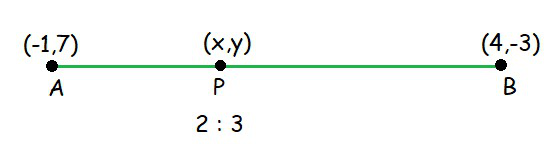where,

m = 2 and n = 3

x1 = -1 and y1 = 7

x2 = 4 and y2 = -3

so, the x coordinate of P will be,

x =x =x =x = 1

and now, the y coordinate of P will be,

y =y =y =y = 3

Hence, the coordinate of P(x,y) is (1,3)

### Question 2. Find the coordinates of the points of trisection of the line segment joining (4, –1) and (–2, –3).

Solution:

Let the point P (x1,y1) and Q(x2,y2) trisects the line.

So, we can conclude that

P divides the line AB in the ratio 1:2.

and Q divides the line AB in the ratio 2:1.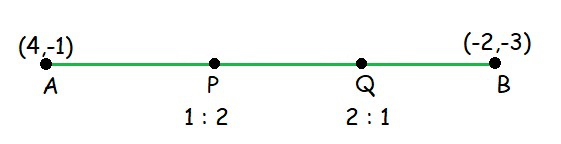• For P

m = 1 and n = 2

x1 = 4 and y1 =-1

x2 = -2 and y2 = -3

so, the x coordinate of P will be,

x =x =x = 2

and now, the y coordinate of P will be,

y =y =y =Hence, the coordinate of P is (2,).

• For Q

m = 2 and n = 1

x1 = 4 and y1 =-1

x2 = -2 and y2 = -3

so, the x coordinate of Q will be,

x =x =x = 0

and now, the y coordinate of Q will be,

y =y =y =Hence, the coordinate of Q is (0,).

### Question 3. To conduct Sports Day activities, in your rectangular shaped school ground ABCD, lines have been drawn with chalk powder at a distance of 1m each. 100 flower pots have been placed at a distance of 1m from each other along AD, as shown in Fig. 7.12. Niharika runs 1/4th the distance AD on the 2nd line and posts a green flag. Preet runs 1/5th the distance AD on the eighth line and posts a red flag. What is the distance between both the flags? If Rashmi has to post a blue flag exactly halfway between the line segment joining the two flags, where should she post her flag?

Solution:

As the given data,

Preet posted red flag atof the distance AD

= (×100) m

= 20m from the starting point of 8th line.

Therefore, the coordinates of this point will be (8, 20).

Similarly, Niharika posted the green flag atth of the distance AD

= (×100) m

= 25m from the starting point of 2nd line.

Therefore, the coordinates of this point will be (2, 25).

Distance between these flags can be calculated by using distance formula,

Distance between two points having coordinates (x1,y1) and (x2,y2) = √((x1-x2)2 + (y1-y2)2)

Distance between these flags = √((8-2)2 + (20-25)2)

= √(62 + 52)

Distance between these flags = √61 m

Now as, Rashmi has to post a blue flag exactly halfway between the two flags. Hence, she will post the blue flag in the mid- point of the line joining these points. where,

m = n =1

(x1,y1) = (8, 20)

(x2,y2) = (2, 25)

x =x =x =x = 5

and now, the y coordinate of Q will be,

y =y =y =y = 22.5

Hence, Rashmi should post her blue flag at 22.5m on 5th line.

### Question 4. Find the ratio in which the line segment joining the points (– 3, 10) and (6, – 8) is divided by (– 1, 6).

Solution:

Lets consider the ratio in which the line segment joining (-3, 10) and (6, -8) is divided by point (-1, 6) be k :1.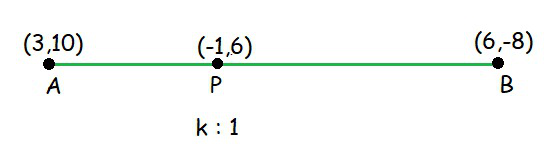m = k and n =1

(x1,y1) = (3, 10) and (x2,y2) = (6,-8)

x = -1

x =-1 =-1(k+1) = 6k+3

k =Hence, the required ratio is 2:7.

### Question 5. Find the ratio in which the line segment joining A(1, – 5) and B(– 4, 5) is divided by the x-axis. Also find the coordinates of the point of division.

Solution:

Let the point P divides the line segment joining A (1, – 5) and B (– 4, 5) in the ratio m : 1.

Therefore, the coordinates of the point of division, say P(x, y) and,

We know that y-coordinate of any point on x-axis is 0.

P(x, 0)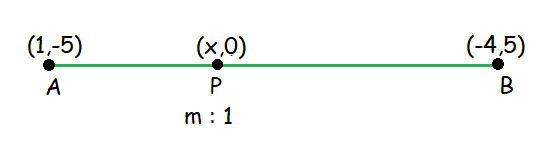m = m and n = 1

(x1,y1) = (1, -5)

(x2,y2) = (-4,5)

so, as the y coordinate of P is 0,

y =0 =5m-5=0

m = 1

So, x-axis divides the line segment in the ratio 1:1.

and, x =x =x =Hence, the coordinate of P is (,0).

### Question 6. If (1, 2), (4, y), (x, 6), and (3, 5) are the vertices of a parallelogram taken in order, find x and y.

Solution:

Let P, Q, R and S be the points of a parallelogram : P(1,2), Q(4,y), R(x,6) and S(3,5).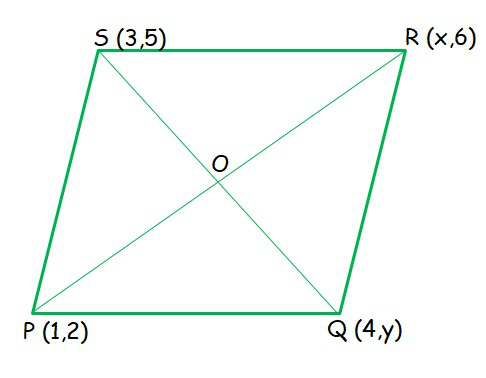Mid point of PR = Mid point of QS (The diagonals of a parallelogram bisect each other, the midpoint O is same)

• Mid point of PR

m = 1 and n = 1

(x1,y1) = (1, 2)

(x2,y2) = (x,6)

so, the x coordinate of O will be,

xoxoxoand now, the y coordinate of O will be,

yoyoyo = 4

So, the coordinate of O is (, 4) ……………..(1)

• For mid point QS

m = 1 and n = 1

(x1,y1) = (3,5)

(x2,y2) = (4,y)

so, the x coordinate of O will be,

xo =xoxoand now, the y coordinate of O will be,

yoyoyoalso , the coordinate of O is……………..(2)

From (1) and (2)and 4 =x = 6 and y = 3

### Question 7. Find the coordinates of a point A, where AB is the diameter of a circle whose centre is (2, – 3) and B is (1, 4).

Solution:

Let the coordinates of point A be (x, y).

Mid-point of AB is C(2, – 3), which is the centre of the circle.

and, Coordinate of B = (1, 4)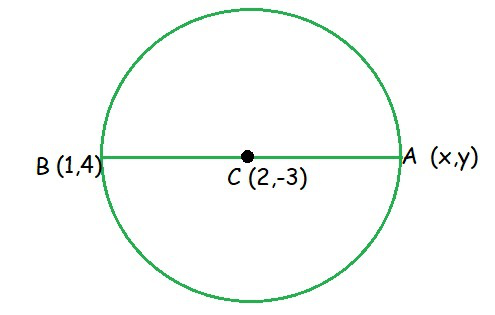For mid point of two points (x1,y1) and (x2,y2)

x =y =By using this formula, we get

(2, -3) == 2 and= -3

x + 1 = 4 and y + 4 = -6

x = 3 and y = -10

The coordinates of A (3,-10).

### Question 8. If A and B are (– 2, – 2) and (2, – 4), respectively, find the coordinates of P such that AP =AB and P lies on the line segment AB.

Solution:

The coordinates of point A and B are (-2,-2) and (2,-4) respectively. Since AP =AB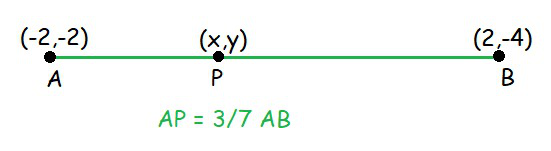=——–(1)

subtract 1 from both sides,– 1 =– 1Therefore, AP: PB = 3:4

Point P divides the line segment AB in the ratio 3:4.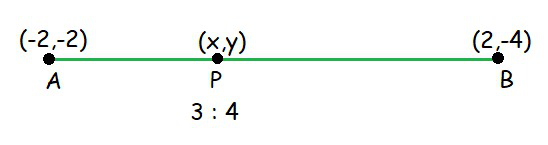Here,

m = 3 and n = 4

(x1,y1) = (-2,-2)

(x2,y2) = (2,-4)

so, the x coordinate of P will be,

x =x =x =and now, the y coordinate of P will be,

y =y =y = \frac{-20}{7}

Hence, the coordinate of P(x,y) is.

### Question 9. Find the coordinates of the points which divide the line segment joining A(– 2, 2) and B(2, 8) into four equal parts.

Solution:

Line segment joining A(– 2, 2) and B(2, 8) divided into four equal parts.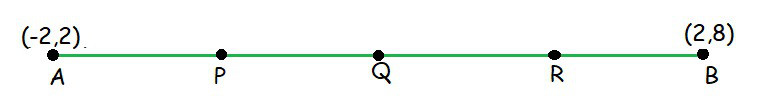• We can say that Q is the mid point of AB

Here,

m = 1 and n = 1

(x1,y1) = (-2,2)

(x2,y2) = (2,8)

so, the x coordinate of Q will be,

x =x =x = 0

and now, the y coordinate of Q will be,

y = \mathbf{\frac{my_2 + ny_1}{m+n}}

y =y = 5

Hence, the coordinate of Q is (0,5)……………………………..(1)

• We can say that P is the mid point of AQ

Here,

m = 1 and n = 1

(x1,y1) = (-2,2)

(x2,y2) = (0,5)

so, the x coordinate of P will be,

x =x =x = -1

and now, the y coordinate of P will be,

y =y =y =Hence, the coordinate of P is (-1,)……………………………..(2)

• Now, we can say that R is the mid point of BQ

Here,

m = 1 and n = 1

(x1,y1) = (2,8)

(x2,y2) = (0,5)

so, the x coordinate of R will be,

x =x =x = 1

and now, the y coordinate of R will be,

y =y =y =Hence, the coordinate of R is (1,)……………………………..(3)

From (1), (2) and (3) we conclude that

Three points between A and B are (-1,), (0,5) and (1,).

### Question 10. Find the area of a rhombus if its vertices are (3, 0), (4, 5), (– 1, 4) and (– 2, – 1) taken in order.[Hint : Area of a rhombus = ½ (product of its diagonals)]

Solution:

Let P(3, 0), Q (4, 5), R(– 1, 4) and S (– 2, – 1) are the vertices of a rhombus PQRS.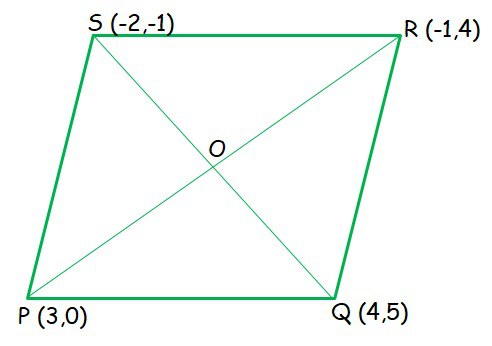Area of a rhombus = ½ (product of its diagonals)

Length of diagonal 1 (PR) = √((3-(-1))2+(0-4)2) = √32 = 4√2 units

Length of diagonal 2 (QS) = √((4-(-2))2+(5-(-1))2) = √72 = 6√2 units

Area of a rhombus = ½ × 4√2 × 6√2

Area of a rhombus = 24 sq. units

Attention reader! Don’t stop learning now. Join the First-Step-to-DSA Course for Class 9 to 12 students , specifically designed to introduce data structures and algorithms to the class 9 to 12 students

My Personal Notes arrow_drop_up